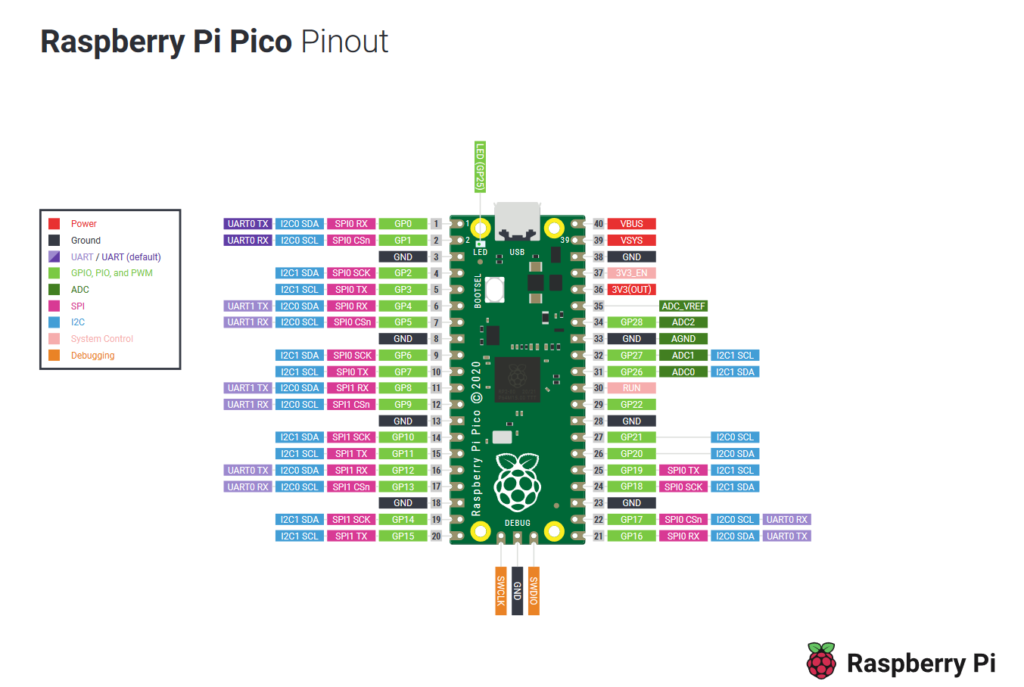Home CodeArduino Get The Temperature from Raspberry Pi Pico

# Get The Temperature from Raspberry Pi Pico

In this tutorial we will read the Temperature from the Raspberry Pi Pico. The Raspberry Pi Pico has an internal Temperature Sensor which is connected to an ADC pin, using Micropython or the Arduino IDE we can read this temperature

The internal temperature sensor that comes with the Raspberry Pi Pico is connected to one of the Analog-to-Digital Converters. The ADC pin supports a range of values, which is determined by the input voltage applied to the pin.

In the Raspberry Pi Pico Board, the ADC pins support 12-bits, which means that the value can go from 0 to 4095.

The MicroPython code though can scale the ADC values to a 16-bit range  which means the range of values that are available are actually 0 to 65535. The microcontroller operates at 3.3 V, this means that an ADC pin will return a value of 65535 at 3.3 V or 0 when there is 0v. So with some simple code we can easily get the value.raspberry pi pico pinout

If you looked at a pinout of the Raspberry pi Pico you may wonder which one of thes pins is actually the ADC pin well the temperature sensor does not connect to a physical pin on the board but is actually ADC4.

### Parts Required

Just a Raspberry Pi Pico required for this one

 Name Link Pico Raspberry Pi Pico Development Board

### Code Examples

2 code example, one for the Arduino IDE and one for Micropython

#### Micropython

[codesyntax lang=”python”]

```import machine
import utime

convert = 3.3 / (65535)

while True:
temperature = 27 - (reading - 0.706)/0.001721
print(temperature)
utime.sleep(1)```

[/codesyntax]

We first convert the raw value to a voltage

[codesyntax lang=”python”]

`convert = 3.3 / (65535)`

[/codesyntax]

From the RP2040 microcontroller datasheet, we see that a temperature of 27 degrees Celsius delivers a voltage of 0.706 V. With each degree the voltage reduces by 1.721 millivolts. Hence we get this formula.

[codesyntax lang=”python”]

`temperature = 27 - (reading - 0.706)/0.001721`

[/codesyntax]

And the output was

13.93637
13.93637
13.93637
14.87265
15.3408

#### Arduino

If you have arduino support installed for the Raspberry Pi Pico then this all you need. We will do a write up of Arduino support in another article. I’m using the one from https://github.com/earlephilhower/arduino-pico

[codesyntax lang=”cpp”]

```void setup()
{
Serial.begin(115200);
delay(5000);
}

void loop()
{
delay(1000);
}```

[/codesyntax]

Open the serial monitor and you should see something like this

Core temperature: 15.9C
Core temperature: 15.9C
Core temperature: 16.8C
Core temperature: 17.3C
Core temperature: 17.8C

#### You may also like

This website uses cookies to improve your experience. We'll assume you're ok with this, but you can opt-out if you wish. Accept Read More